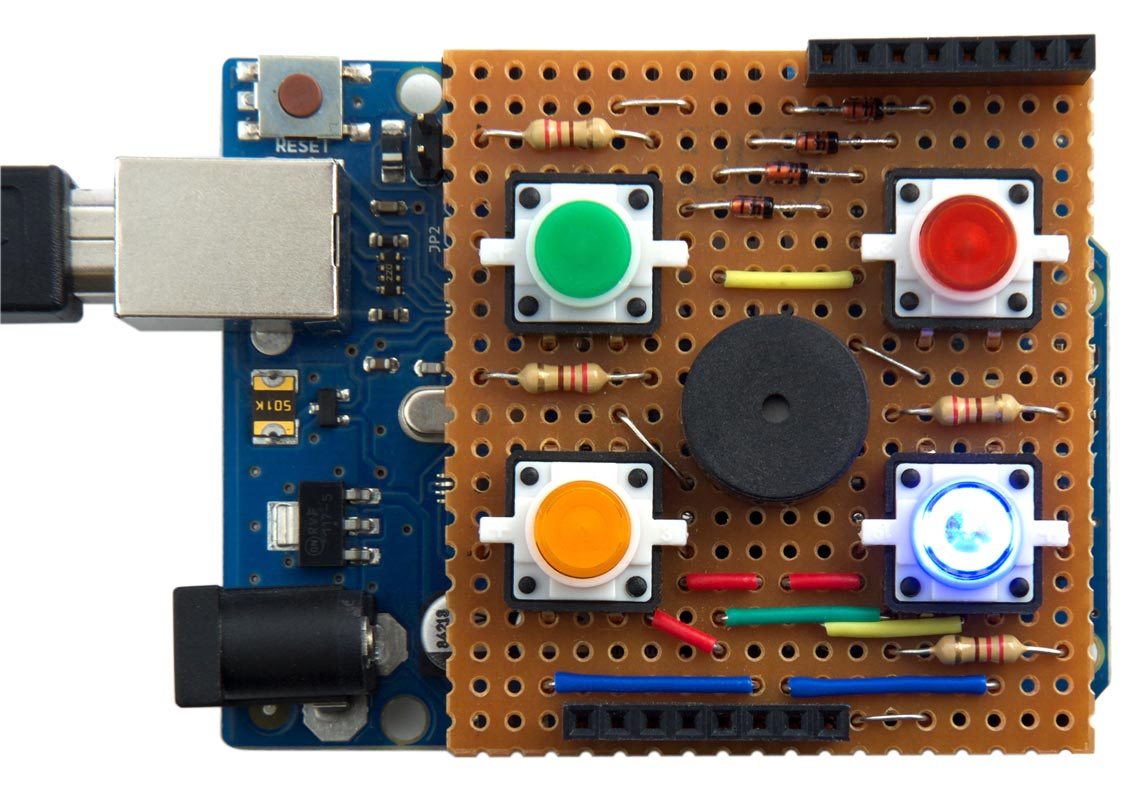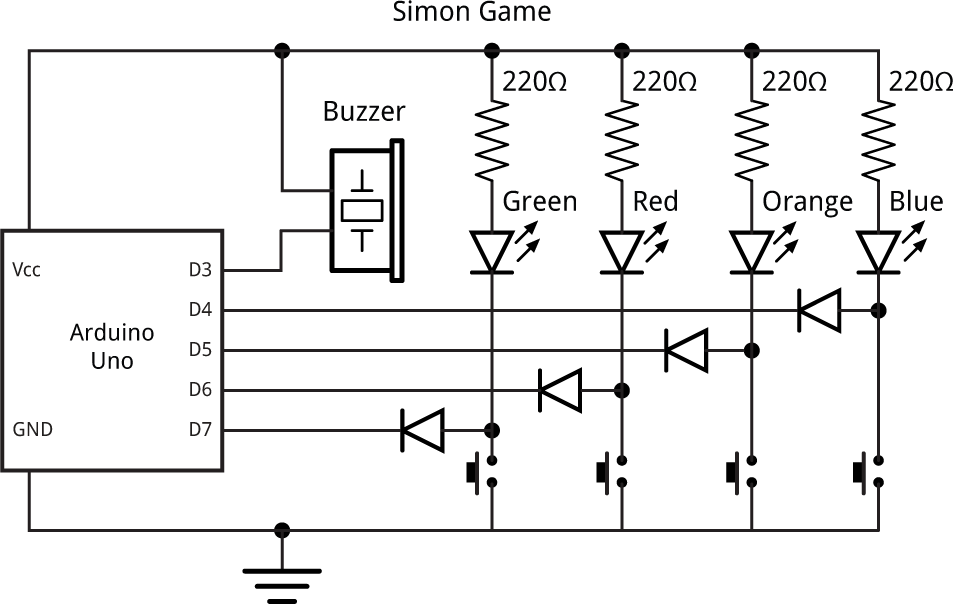## Simon game

To illustrate what can be achieved with uLisp running on an Arduino Uno, or ATmega328, here's a program to play the Simon game, using a circuit of four buttons, four LEDs, and a piezo speaker.

If you're not familiar with it, the game plays a sequence of tones and lights, and you have to press the correct buttons to reproduce the sequence. At each round the sequence increases in length, and the aim is to reproduce the longest possible sequence without making a mistake.

### The Simon shield

The game uses a shield for the Arduino that provides four buttons, four coloured LEDs, and a piezo speaker:Shield for the Arduino Uno to let you play Simon programmed in uLisp.

I used illuminated coloured push buttons with integral LEDs, available from Sparkfun , but you could alternatively use separate push buttons and coloured LEDs. The shield was built on a square of veroboard, with header plugs designed to fit into the sockets on the Arduino Uno.

### The circuit

The circuit uses just four lines to control the LEDs and read the pushbuttons:Circuit of the Simon shield for the Arduino Uno.

As well as saving I/O lines, this circuit automatically lights up the corresponding LED when you press a button. To read the status of a push button program the I/O line as an input, and to light a LED program the line as an output and leave it low.

The four diodes are not strictly necessary, but protect each I/O line in case the line is inadvertently programmed as an output and taken high while the push button is pressed. They can be low cost signal diodes such as the 1N4148.

### The uLisp program

Here's the program to run the Simon game. First pns defines a list of the Arduino pins used for the buttons and LEDs:

`(defvar pns '(4 5 6 7))`

The function del gives a delay of 250 microseconds:

`(defun del () (delay 250))`

Next, bep flashes an LED and plays the corresponding note of the correct frequency:

```(defun bep (x)
(let* ((p (nth x pns)))
(pinmode p t)
(note 3 (nth x '(52 49 57 40)))
(del)
(note)
(pinmode p nil)
(del)))```

This calls the following uLisp note function to actually play the note through pin D3:

`(note 3 (nth x '(52 49 57 40)))`

The notes are :

• E 4 (blue, lower right),
• C# 4 (yellow, lower left),
• A 4 (red, upper right),
• E 3 (green, upper left).

The note function is called with no arguments to silence the note.

The function chk waits until the corresponding button is pressed, and then plays the appropriate note by calling bep:

```(defun chk (x)
(loop
(unless (digitalread (nth x pns)) (bep x) (return))))```

You can test the functions pn, bep, and chk interactively by evaluating them with an argument from 0 to 3; for example:

`> (bep 2)`

Finally, sim runs the complete Simon game:

```(defun sim ()
(let* ((seq (list (random 4))))
(loop
(mapc bep (reverse seq))
(mapc chk (reverse seq))
(push (mod (+ (car seq) (random 3) 1) 4) seq)
(del))))```

For each new sequence it calls bep to play and flash the sequence, and then calls chk to wait for the correct sequence of button presses. The line:

`(push (mod (+ (car seq) (random 3) 1) 4) seq)`

adds a random number onto the front of the sequence, making sure that it differs from the previous number.

On an ATmega328 the game can play with a sequence of about 12 notes before the program runs out of room.

Here's the full program for the Simon game: Simon game program.

1. ^ LED Tactile Button - Red on SparkFun.
2. ^ Simon (game) on Wikipedia